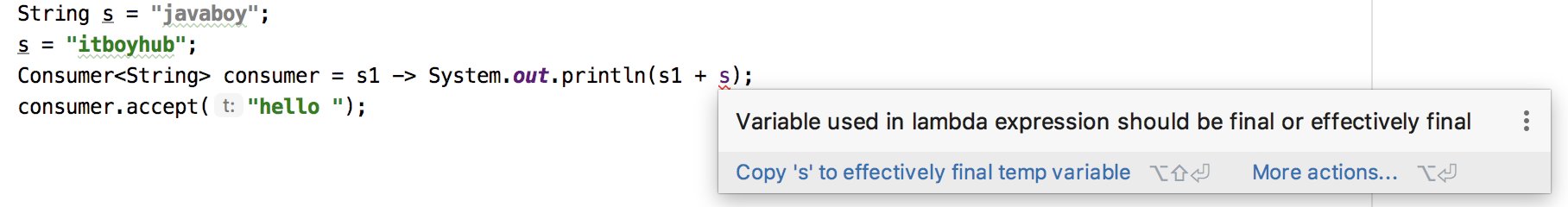# WebFlux 前置知识（二）

## 1 方法引用

### 2.2 四种方法引用

#### 2.2.1 静态方法引用

public class LambdaDemo05 {
public static void main(String[] args) {
Function<Integer, String> func = a -> String.valueOf(a);
String s = func.apply(99);
System.out.println("s = " + s);
}
}


public class LambdaDemo05 {
public static void main(String[] args) {
Function<Integer, String> func = String::valueOf;
String s = func.apply(99);
System.out.println("s = " + s);
}
}


Consumer<String> consumer = s -> System.out.println(s);
consumer.accept("javaboy");


Consumer 消费一个字符串，消费的方式就是控制台打印，这种时候我们可以简写成如下方式：

Consumer<String> consumer = System.out::println;
consumer.accept("javaboy");


BiFunction<Integer, Integer, Double> func = (a, b) -> Math.pow(a, b);
Double result = func.apply(3, 4);
System.out.println("result = " + result);


BiFunction<Integer, Integer, Double> func = Math::pow;
Double result = func.apply(3, 4);
System.out.println("result = " + result);


#### 2.2.2 实例方法引用

Random random = new Random();
IntUnaryOperator func = i -> random.nextInt(i);
Integer r = func.applyAsInt(10);
System.out.println("r = " + r);


Random random = new Random();
IntUnaryOperator func = random::nextInt;
Integer r = func.applyAsInt(10);
System.out.println("r = " + r);


String[] stringArray = {"Barbara", "Mary", "James"};
Arrays.sort(stringArray,String.CASE_INSENSITIVE_ORDER);
System.out.println(Arrays.toString(stringArray));


String[] stringArray = {"Barbara", "Mary", "James"};
Arrays.sort(stringArray, String::compareToIgnoreCase);
System.out.println(Arrays.toString(stringArray));


#### 2.2.3 构造方法引用

Supplier<Cat> supplier = () -> new Cat();
Cat cat = supplier.get();


Supplier<Cat> supplier = Cat::new;
Cat cat = supplier.get();


#### 2.2.4 数组构造方法引用

IntFunction<int[]> func = (i) -> new int[i];
int[] arr = func.apply(10);
System.out.println("arr.length = " + arr.length);


IntFunction<int[]> func = int[]::new;
int[] arr = func.apply(10);
System.out.println("arr.length = " + arr.length);


## 3. 变量引用

String s = "javaboy";
Consumer<String> consumer = s1 -> System.out.println(s1 + s);
consumer.accept("hello ");## 4. 类型推断

@FunctionalInterface
interface ICalculator2{
}
@FunctionalInterface
interface ICalculator3{
int multiply(int a, int b);
}
public static void main(String[] args) {
calculator((ICalculator2) (a, b) -> a + b);
}

public static void calculator(ICalculator2 iCalculator) {
}
public static void calculator(ICalculator3 iCalculator) {
}
}


## 5. 级联表达式

Lambda 表达式也可以写成 N 多层，具体则看需求。

Function<Integer, Function<Integer, IntFunction<Integer>>> func = x -> y -> z -> x + y + z;
Integer i = func.apply(3).apply(4).apply(5);
System.out.println("i = " + i);


z->x+y+z 对应的是 IntFunction<Integer>

z->x+y+z 整体作为返回，y 作为输入，对应的是 Function<Integer, IntFunction<Integer>>

y -> z -> x + y + z 整体作为返回，x 作为输入，对应的就是 Function<Integer, Function<Integer, IntFunction<Integer>>>

that’s all。

• https://blog.csdn.net/sun_promise/article/details/51190256
• https://www.jianshu.com/p/4a3da6a11b58• 微信咨询